# S6E2 - How to Calculate Internal Energy, Work, and Heat

Two key definitions before we begin:

- thermodynamics = the study of energy and its interconversions.

- 1st Law of Thermodynamics = the total energy of the universe is constant.

=====

## The Internal Energy of a System (E)

- think of the "system" as the "chemical reaction."

The Internal Energy of a system - the sum of the kinetic and potential energies of all the "particles" in the system (the reaction).

➞ this internal energy (E) can be changed by a flow of work (w), heat (q), or both:

### ΔE  =  q  +  w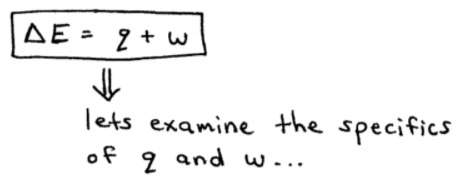As the image above suggests, let's examine the specifics of heat (q) and work (w)...

... the signs (+ or -) of heat (q) and work (w) are identified from the point of view of the system:

A. if the reaction is endothermic, heat flows into the system and thus heat (q) is positive (+) q > 0

B. if the reaction is exothermic, heat flows out of the system and thus heat (q) is negative (-) q < 0

-----

C. if the reaction (system) does work (w) on the surroundings, energy flows out of the system, so work (w) is negative (-) w < 0

D. if the surroundings do work (w) on the system, energy flows into the system, so work (w) is positive (+) w > 0

-----

ex:  Calculate ΔE for a system undergoing an endothermic process in which 15.6 kJ of heat flows and where 1400 J of work is done on the system.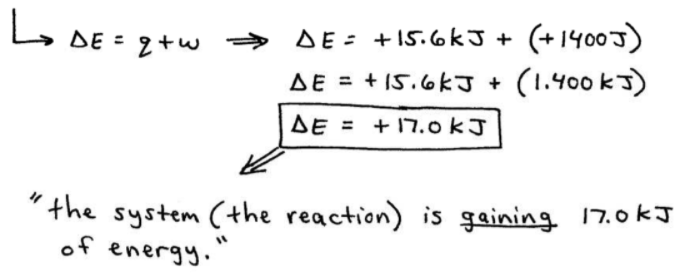=====

## How to Calculate Work (w)

The "work" associated with chemical processes is usually work done by gases (through expansion) or work done to gases (through compression).

Let's examine 2 CASES (below) and the following relationship between work, external pressure, and volume:  w = - PΔV

- external pressure (P) = usually a constant in these types of problems, so w depends on ΔV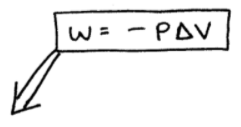CASE 1 ➞ If a gas expands, the volume of the system increases, so the change in volume (ΔV) is positive (+).

Because pressure (P) is always positive (+), then the work (w) must be negative (-).

➞ this corelates well with "C" above because the reaction is doing work on the surroundings in order to expand the volume.

-- so, w < 0 (-) because energy is flowing out of the system.

CASE 2 ➞ If a gas gets compressed, the volume of the system decreases, so the change in volume (ΔV) is negative (-).

Because pressure (P) is always positive (+), then according to "w = -PΔV", the work (w) must be positive (+).

➞ this corelates well with "D" above because the surroundings are doing work on the system in order to compress the volume.

-- so, w > 0 (+) because energy is flowing into the system.

=====

ex:  Calculate the work associated with the expansion of a gas from 46L to 64L at a constant external pressure of 15atm.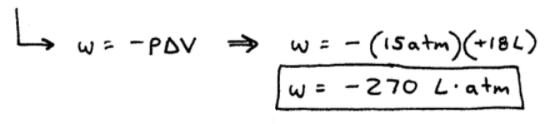This is an "expansion" (CASE 1).

Don't think of the (-) minus sign as a "negative number," but instead think of the (-) sign as a direction.

➞ since the gas expands, work is negative (case 1).

-----

ex:  A balloon is being inflated by heating the air inside it. The volume of the balloon increases from 4.00 x 106 L to 4.50 x 106 L by the addition of 1.3 x 108 J of energy as heat.

Assuming that the balloon expands against a constant pressure of 1.0atm, calculate ΔE for this process. (note: 1 L.atm = 101.3 J )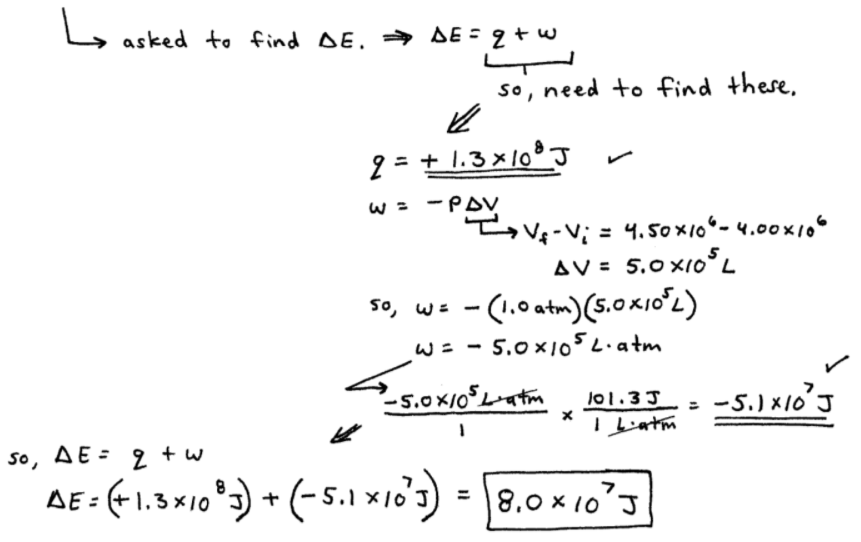=====

Next, we'll talk about how "heat" (q) is measured experimentally using a concept called Calorimetry.

So, stick around for my next post on SECTION 6 - Thermochemistry,

where we'll discuss Calorimetry and Specific Heat Capacity...